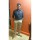Java Developers
Group for Java developers and programmers. Ask questions and get answers related to Java programming
254 Members
Join this group to post and comment.DHIRAJ LOTLIKAR
Information Technology
06 Feb 2019

# What is the initial capacity and load factor of Hashmap in Java?

HashMap is one of the high performing data structure in java collection framework. Whatever may be the size of the data, HashMap almost gives constant time performance for most frequent operations – insertion and retrieval. That’s why HashMap is the first choice for the big sized data having requirement of faster retrieval and faster insertion operations.

There are two factors which affect the performance of HashMap. One is the load factor and another one is initial capacity. You have to choose these two factors very carefully while constructing an HashMap object. In this post, we will have a look at initial capacity and load factor in HashMap and see how they affect the performance of HashMap.

### Initial Capacity Of HashMap :

The capacity of an HashMap is the number of buckets in the hash table. The initial capacity is the capacity of an HashMap at the time of its creation. The default initial capacity of the HashMap is 24 i.e 16.

The capacity of the HashMap is doubled each time it reaches the threshold. i.e the capacity is increased to 25=32, 26=64, 27=128….. when the threshold is reached.

Load factor is the measure which decides when to increase the capacity of the HashMap. The default load factor is 0.75f.

Threshold of Hashmap:

The threshold of an HashMap is the product of current capacity and load factor.

Threshold = (Current Capacity) * (Load Factor)

For example, if the HashMap is created with initial capacity of 16 and load factor of 0.75f, then threshold will be,

Threshold = 16 * 0.75 = 12

That means, the capacity of the HashMap is increased from 16 to 32 after the 12th element (key-value pair) is added into the HashMap